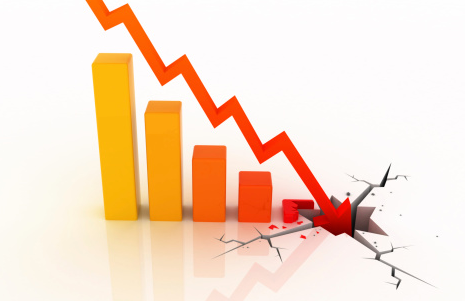## Straight Line Depreciation

A machine shop purchases a piece of equipment that costs $8,000. It has a salvage value of$500, and a 10 year lifespan. Calculate the straight line depreciation.Hint
The straight line depreciation formula is:
$$D_{j}=\frac{C-S_{n}}{n}$$$where $$C$$ is the initial cost, $$n$$ is the life span, and $$S_n$$ is the expected salvage value in year $$n$$ . Hint 2 The answer should be in dollars per year. The straight line depreciation formula is: $$D_{j}=\frac{C-S_{n}}{n}$$$
where $$C$$ is the initial cost, $$n$$ is the life span, and $$S_n$$ is the expected salvage value in year $$n$$ .
$$D_{j}=\frac{\8,000-\500}{10\:year}=\750/yr$$$The equipment is depreciating$750 every year.
\$750 per year